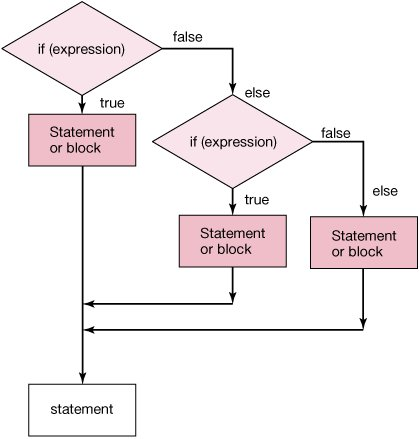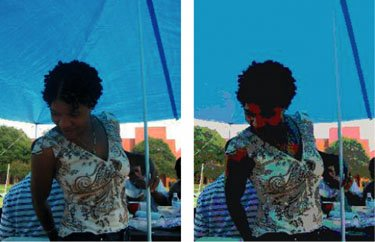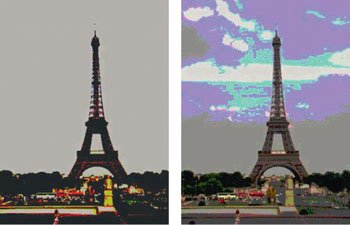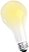# Section 6.3. Sepia-Toned and Posterized Pictures: Using Multiple Conditionals to Choose the Color

[Page 186 (continued)]

### 6.3. Sepia-Toned and Posterized Pictures: Using Multiple Conditionals to Choose the Color

We handled the case of having two different ways to process the pixels using an if and else. What if we have more than two ways that we want to process some pixels? For example, what if we wanted to do one thing if a value is less than some number, another thing if it is equal and yet a third if it is greater than the number? We could check for each of these conditions with an if as shown below:

`> int y = 10; > if (y < 10) System.out.println("y is less than 10"); > if (y == 10) System.out.println("y is equal to 10"); y is equal 10 > if (y > 10) System.out.println("y is greater than 10");`

This works but results in some unnecessary checking. Notice that y was equal to 10 and so that was printed out but it still executed the next statement which checked if y was greater than 10. But, can y be equal to 10 and greater than 10? What would have happened if y was less than 10? It would have printed out a string saying that y is less than 10 and then still checked if y was equal or greater than 10. We need something to say that if the previous test was true, execute that and then skip to the end of all the checks. We have seen a way to do this for two possibilities (true or false) using if and else. One way to handle three or more possibilities is with if, else if and else (Figure 6.8). You can use as many else if statements as needed. You are not required to have a final else.

`> int y = 2; > if (y < 10) System.out.println("Y is less than 10");   else if (y == 10) System.out.println("y is equal to 10");   else System.out.println("y is greater than 10"); y is less than 10`

##### Figure 6.8. Flowchart of an if, else if, and an else. (This item is displayed on page 187 in the print version)So far, we've done color modification by simply saying "This color replaces that color." We can be more sophisticated in our color swapping. We can look for a range of colors, by using if, else if, and else, and replace the color with some function of the original color or a specific color. The results are quite interesting.

For example, we might want to generate sepia-toned prints. Older prints sometimes have a yellowish tint to them. We could just do an overall color change, but the end result isn't aesthetically pleasing. By looking for different kinds of colorhighlights, middle ranges, and shadowsand treating them differently, we can get a better effect (Figure 6.9).

[Page 187]

##### Figure 6.9. Original scene (left) and using our sepia-tone program.The way we do this is to first convert the picture to gray, both because older prints were in shades of gray, and because it makes it a little easier to work with. We then look for high, middle, and low ranges of color, and change them separately. We want to make the shadows (darkest grays) a bit darker. We want to make most of the picture (middle grays) into a brownish color. We want to make the highlights (lightest grays) a bit yellow. Recall that yellow is a mixture of red and green, so one way to make things yellow is to increase the red and green. Another way is to reduce the amount of blue. The advantage to reducing the blue is that you don't have to worry about increasing a value past 255 which is the maximum.

[Page 188]
##### Program 38. Convert a Picture to Sepia-Tones (This item is displayed on pages 188 - 189 in the print version)`/** * Method to change the current picture to a sepia * tint (modify the middle colors to a light brown and * the light colors to a light yellow and make the * shadows darker) */ public void sepiaTint() { Pixel pixel = null; double redValue = 0; double greenValue = 0; double blueValue = 0; // first change the current picture to grayscale this.grayscale(); // loop through the pixels for (int x = 0; x < this.getWidth(); x++) { for (int y = 0; y < this.getHeight(); y++) { // get the current pixel and color values pixel = this.getPixel(x,y); redValue = pixel.getRed(); greenValue = pixel.getGreen(); blueValue = pixel.getBlue(); // tint the shadows darker if (redValue < 60) { redValue = redValue * 0.9; greenValue = greenValue * 0.9; blueValue = blueValue * 0.9; } // tint the midtones a light brown // by reducing the blue else if (redValue < 190) { blueValue = blueValue * 0.8; } // tint the highlights a light yellow // by reducing the blue else [Page 189] { blueValue = blueValue * 0.9; } // set the colors pixel.setRed((int) redValue); pixel.setGreen((int) greenValue); pixel.setBlue((int) blueValue); } } }`

Try this method out by:

`> Picture picture = new Picture(Picture.getMediaPath("gorge.jpg")); > picture.show(); > picture.sepiaTint(); > picture.repaint();`

Posterizing is a process of converting a picture to a smaller number of colors. We're going to do that by looking for specific ranges of color, then setting the color to one value in that range. The result is that we reduce the number of colors in the picture (Figure 6.10).

##### Figure 6.10. Reducing the colors (right) from the original (left).[Page 190]

##### Program 39. Posterizing a Picture (This item is displayed on pages 190 - 191 in the print version)`/** * Method to posterize (reduce the number of colors) in * the picture. The number of reds, greens, and blues * will be 4. */ public void posterize() { Pixel pixel = null; int redValue = 0; int greenValue = 0; int blueValue = 0; // loop through the pixels for (int x = 0; x < this.getWidth(); x++) { for (int y = 0; y < this.getHeight(); y++) { // get the current pixel and colors pixel = this.getPixel(x,y); redValue = pixel.getRed(); greenValue = pixel.getGreen(); blueValue = pixel.getBlue(); // check for red range and change color if (redValue < 64) redValue = 31; else if (redValue < 128) redValue = 95; else if (redValue < 192) redValue = 159; else redValue = 223; // check for green range if (greenValue < 64) greenValue = 31; else if (greenValue < 128) greenValue = 95; else if (greenValue < 192) greenValue = 159; else greenValue = 223; // check for blue range if (blueValue < 64) blueValue = 31; else if (blueValue < 128) blueValue = 95; else if (blueValue < 192) blueValue = 159; [Page 191] else blueValue = 223; // set the colors pixel.setRed(redValue); pixel.setGreen(greenValue); pixel.setBlue(blueValue); } } }`

What's really going on here, though, is setting up (a) a bunch of levels, then (b) setting the value of red, green, or blue to the midpoint of that level. We can do this more generally using mathematics to compute the ranges for a desired number of levels and picking the midpoint. We need to check whether the current value is in the range and if so set it to the midpoint of the range.

How do we check whether a value is in a range? If we call the bottom of the range bottomValue and the top of the range topValue, then we could use this math notation bottomValue <= testValue < topValue. However in Java we need to write it bottomValue <= testValue && testValue < topValue. The two ampersands ('&&') mean 'and'. If your mother says that you have to set the table and sweep the floor, how many jobs do you have to do? The answer is two, or both of them. If she says that you can set the table or sweep the floor, how many jobs do you have to do then? The answer is one, or just one of the two. Similarly if in Java you have if (expression && expression) then both expressions must be true for the body of the if to be executed. And, if you have if (expression || expression) then only one of the two expressions must be true for the body of the if to be executed. The || means 'or'.

Below is the program for a flexible number of levels, and Figure 6.11 shows a couple of examples.

##### Figure 6.11. Pictures posterized to two levels (left) and four levels (right). (This item is displayed on page 192 in the print version)##### Program 40. Posterize by Levels (This item is displayed on pages 191 - 192 in the print version)`/** * Method to posterize (reduce the number of colors) in * the picture * @param numLevels the number of color levels to use */ public void posterize(int numLevels) { Pixel pixel = null; int redValue = 0; int greenValue = 0; int blueValue = 0; int increment = (int) (256.0 / numLevels); int bottomValue, topValue, middleValue = 0; // loop through the pixels for (int x = 0; x < this.getWidth(); x++) { for (int y = 0; y < this.getHeight(); y++) { [Page 192] // get the current pixel and colors pixel = this.getPixel(x,y); redValue = pixel.getRed(); greenValue = pixel.getGreen(); blueValue = pixel.getBlue(); // loop through the number of levels for (int i = 0; i < numLevels; i++) { // compute the bottom, top, and middle values bottomValue = i * increment; topValue = (i + 1) * increment; middleValue = (int) ((bottomValue + topValue - 1) / 2.0); /* check if current values are in current range and * if so set them to the middle value */ if (bottomValue <= redValue && redValue < topValue) pixel.setRed(middleValue); if (bottomValue <= greenValue && greenValue < topValue) pixel.setGreen(middleValue); if (bottomValue <= blueValue && blueValue < topValue) pixel.setBlue(middleValue); } } } }`

[Page 193]Notice that we now have two methods called posterize. One method doesn't take any parameters, and the other that takes the number of levels. In Java you are allowed to have more than one method with the same name as long as the parameters are different. This is called overloading. You can have a different number of parameters as in this case. You can also use different types and/or orders for the parameters. It means that you can have several methods that have the same behavior but operate on different parameters. It certainly is easier than coming up with new names for methods that do that same thing.Introduction to Computing & Programming Algebra in Java(c) A Multimedia Approach
ISBN: N/A
EAN: N/A
Year: 2007
Pages: 191